# Methods for Scoring Words in NLP

Natural language processing is defined as the interaction between the computer and the human language.

As we all are aware, that the human language is messy, and there are a lot of different ways of saying the same thing.

There are a lot of ways in which human beings can communicate with each other, but what about the communication of humans and computers??

That is where Natural Language Processing comes in.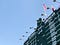Photo by Jason Weingardt on Unsplash

While solving problems related to NLP, textual data is converted into numerical data for the machine to understand. This conversion is very crucial to the results of an NLP…

# Create simple Bag-of-Words models

In the previous blog of the series, we read about what a Bag-of-Words model is, and how we can manually create a simple model.

If you haven't read the blog, read it here: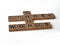Photo by Brett Jordan on Unsplash

In this blog, we will learn how to create simple BoW models using Scikit-Learn and Keras.

# Create BoW using Scikit-Learn

There are different types of scoring methods that can be used to convert textual data to numerical vectors. You can read about these techniques here.

Scikit-Learn provides different methods for the conversion of textual data into vectors of numerical values. Two of these methods are:

• CountVectorizer
• TfidfVectorizer

## CountVectorizer

Convert a collection of…

# Bag-of-Words models in NLP

In this 2 part series, we shall see how we can develop a simple Bag-of-Words Model in Natural Language Processing.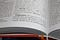Photo by Stefan on Unsplash

We shall start by understanding what the Bag-Of-Words model is, how we can develop a simple model using Scikit-Learn and keras.

# What is the Bag-Of-Words model?

Broadly speaking, a bag-of-words model is a representation of text which is usable by the machine learning algorithms. As we all know, most machine learning algorithms cannot work directly with non-numerical data, which is why we use various encoding methods like One-Hot-Encoding to convert this textual data into numerical matrices, to be used by the algorithm.

Bag-Of-Words (BoW) aims…

# Vanishing Gradient Descent Problem In-Depth

Vanishing gradient descent was a big problem while training neural network models with more layers. This is because, the addition of more layers to a network, led to the vanishing of the weight values, thereby resulting in almost constant values for computation. We say that the values are almost constant because the change is not significant.

Let us understand this by looking into the problem in-depth.

## What is Vanishing Gradient Descent

The decrease of the backpropagated error signal (often exponentially) with the increasing distance from the final layer (typically the output layer) is the problem pointing to Vanishing Gradient Descent.

But what does this mean…

# How Perceptrons solve the linearly separable problems

A perceptron is a single neuron model that may be a forerunner to a large network.

Perceptrons perform the computations to output binary values 0 or 1 (the output values can also be -1 and 1 depending on the activation function used).

## What is a linearly separable problem?

Linear separability is a property of two sets of points. This is most easily visualized in two dimensions (the Euclidean plane) by thinking of one set of points as being colored blue and the other set of points as being colored red. …

# What is Artificial Neural Network

With the growing need for better ways to train a machine to understand on its own, people have been taking inspiration from the animal brain to train the machine in a way such that it is able to understand literally anything on its own.

This is where the concept of Artificial Neural Networks (ANN) comes in. What if the machines could imitate the animal brain to learn from the information presented before it.

As per the definition of ANN,

An artificial neural network (ANN) is the component of artificial intelligence that is meant to simulate the functioning of a human…

# Solving the XOR problem using MLP

In this blog post, we shall cover the basics of what the XOR problem is, and how we can solve it using MLP.

What is XOR?

Exclusive or is a logical operation that outputs true when the inputs differ.

For the XOR gate, the TRUTH table will be as follows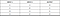XOR truth table

XOR is a classification problem, as it renders binary distinct outputs. If we plot the INPUTS vs OUTPUTS for the XOR gate, it would look something like

# Data Preparation in Machine Learning

Data preparation is a very important aspect of Machine Learning. This is vital to any Machine learning project for a variety of reasons. We shall discuss these reasons and some ways by which we can prepare our data for our models.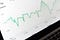Photo by Markus Winkler on Unsplash

# What is Data Preparation?

Data preparation is the process by which we clean and transforms the data, into a form that is usable by our Machine Learning project. In this process, raw data is transformed for better understanding and analysis.

## But why do we need to prepare the data?

The biggest reason behind data preparation is that the algorithms that are used in Machine learning mostly use numerical inputs. …

# Introduction to Vector Norms

Vector calculations are important either directly or as a method that makes slight modifications to the learning algorithm such that the model generalizes better.

Vectors are used throughout the field of Machine Learning while formulating algorithms and processes to achieve the optimal target variable (y) after the training of the algorithm.

# What is vector norm?

Vector matrix operations often require you to calculate the length (or size) of a vector. In two dimensional space, the length of a vector is defined as,

The square root of the sum of the squares of the horizontal and vertical components.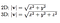Length of vector for 2D and 3D space.

The length of a vector is what…Android | AI